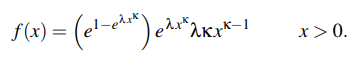# Exponential Power Distribution: Definition, PDF

The exponential power distribution is a generalization of the normal distribution. Therefore, it’s also known as the generalized normal distribution. Other names include the General Error Distribution and the Generalized Power Distribution.

## PDF of the Exponential Power Distribution

The Probability Density Function (PDF) of the Exponential Power Distribution is:Where:

The extra parameter allows the distribution to represent many more distributional shapes. It also allows more flexibility with kurtosis, which can be represented by a variety of values (rather than just a constant).

As well as the extra parameter, which allows more flexibility with distributional shapes and kurtosis. However, a “major drawback” is that the distribution doesn’t allow for asymmetrical data (Konunjer, n.d.). However, it is possible to derive a slightly different model with skew (e.g. the skew exponential power model proposed by Ayebo and Kozubowski).

Other features include:

## References

Ayebo, A. and Kozubowski, T. An asymmetric generalization of Gaussian and Laplace laws. Retrieved Auhust 12, 2019 from: https://wolfweb.unr.edu/homepage/tkozubow/0skeexp1.pdf
Package Normalp. Retrieved August 12, 2019 from: https://cran.case.edu/web/packages/normalp/normalp.pdf
Exponential Power. Retrieved August 12, 2019 from: http://www.math.wm.edu/~leemis/chart/UDR/PDFs/Exponentialpower.pdf
Konunjer, I. Asymmetric Power Distribution: Theory and Applications to Risk Measurement.
Kotchlamazashvili, Z. (2014). Empirical Analysis of the EU Term Structure of Interest Rates. Logos Verlag Berlin GmbH.

CITE THIS AS:
Stephanie Glen. "Exponential Power Distribution: Definition, PDF" From StatisticsHowTo.com: Elementary Statistics for the rest of us! https://www.statisticshowto.com/exponential-power-distribution/